Select Page

## Did you try each several times?

Here are the answers to Brain Practice, November, 2019

#### Complete the pattern.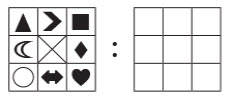#### Words Puzzle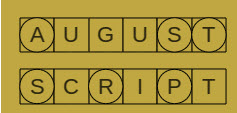Word from the first row: AUGUST
Word from the second row: SCRIPT

Word from the circled letters: STRAPS or SPRATS.

Did you have a different solution?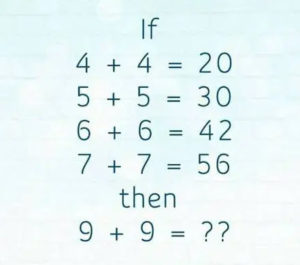SOLUTION :-
IF,  4*4 = 20              ( 4×4 + 4 = 20 )
5*5 = 30                      ( 5×5 + 5 = 30 )
6*6 = 42                      ( 6×6 + 6 = 42 )
7*7 = 56                       ( 7×7 + 7 = 56 )
8*8 = 72  ( MISSING Number )          ( 8*8 + 8 = 72 )

SO ,  9*9 = 90            ( 9×9 + 9 = 90 )

#### How many -ologies did you know?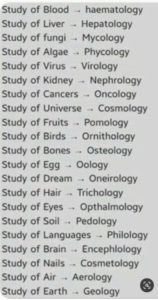Study of the Brain can also be Neurology.

Any other differences??

#### Did you Solve these?

I am a three digit number.
My tens digit is five more than my ones digit.
My hundreds digit is eight less than my tens digit.
What number am I?
Thank you to Dave C. and Mike B. who provide this reasoning:

Ones digit calc is 4
Tens 5 more than ones =4+5 = 9
Hundreds 8 less than tens =9-8= 1

So the answer is NOT 194 – it is 491. Thank you dave and Mike!

—————
Peter owns a pet store. He puts one canary per cage but has one bird too many. If he puts two canaries per cage he has one cage too many. How many canaries and cages does Peter have?
Peter has 3 cages and 4 canaries.
—————
Why did the golfer take two pairs of pants to the course? In case he got a hole in one. (Sorry! That is corny ….)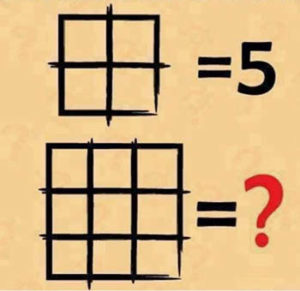The answer is 14.

All the little squares (9) + There are 4 bigger squares (made up of 4 little squares) + the one big square.

9 + 4 + 1 = 14